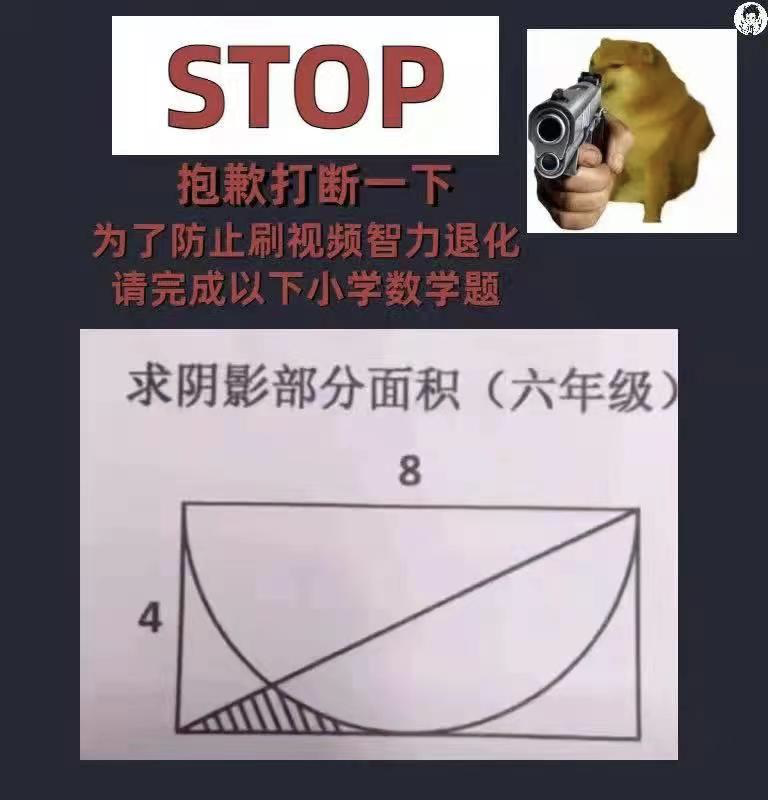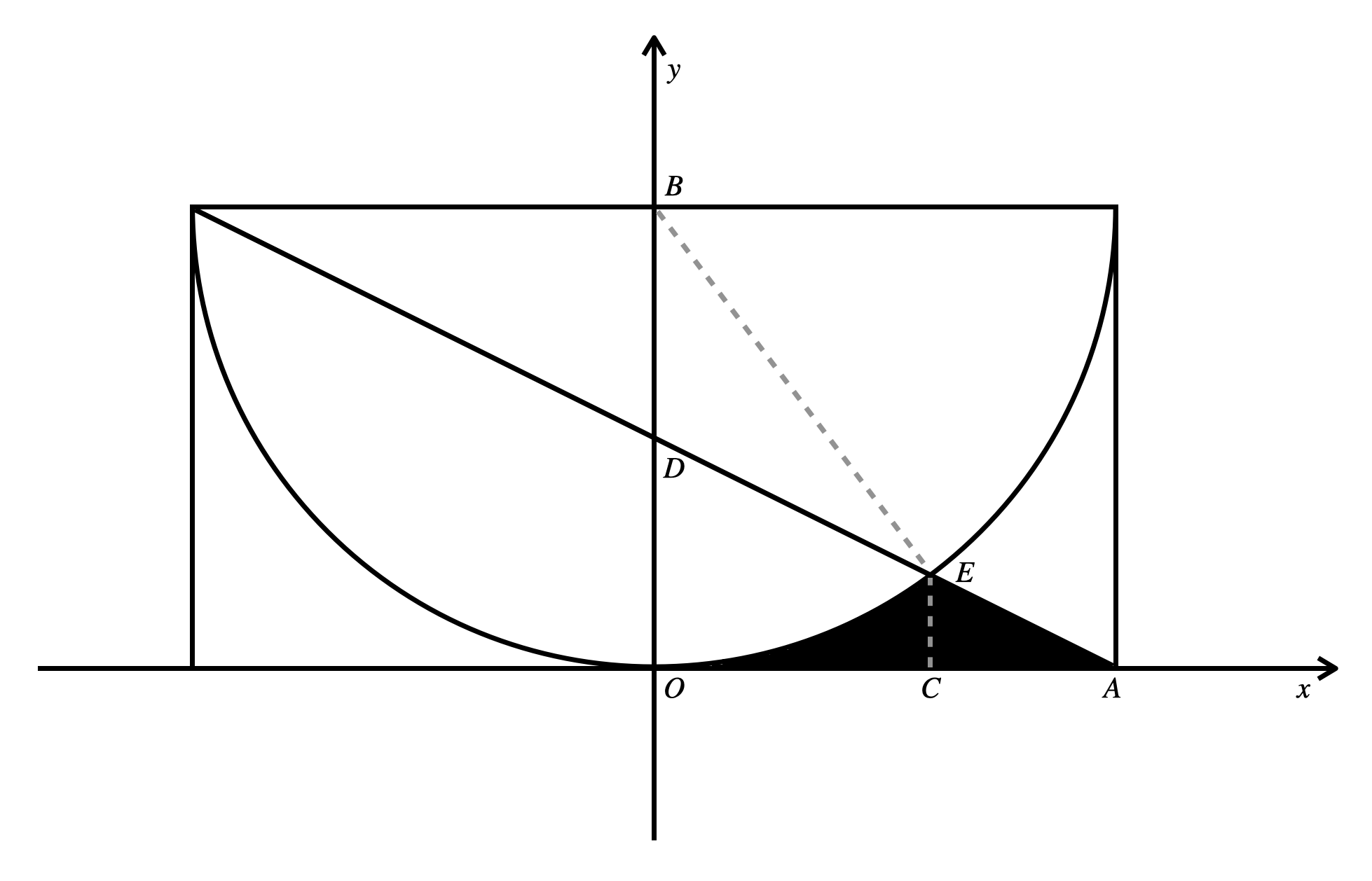## 积分方法

\begin{aligned} x^2+(y-4)^2-16&=0 \\ x+2y-4&=0 \end{aligned}

$$\int_{0}^{\frac{12}{5}}{\left(\sqrt{16-x^2}+4\right)}dx + \int_{\frac{12}{5}}^{4}{\left(2-\frac{1}{2}x\right)}dx$$

$$\frac{32}{5} - 8 \arcsin{\frac{3}{5}}$$

## 蒙特卡洛采样方法

### C++实现

#include <iostream>

typedef unsigned long long int ull;

int main() {
srand(time(NULL));
ull res = 0;
ull cnt = 1e8;
for (ull i = 0; i < cnt; ++i) {
double x = double(rand()) / RAND_MAX;
double y = double(rand()) / RAND_MAX;
if (x * x + (y - 1) * (y - 1) > 1 && x + 2 * y - 1 < 0) {
res += 1;
}
}
printf("%.5f\n", res * 16.0 / cnt);
return 0;
}

### CUDA实现

#include <iostream>
#include <chrono>
#include <curand_kernel.h>

typedef unsigned long long int ull;

__global__ void monte_carlo_kernel(ull* res, ull cnt, const int seed) {
ull i = blockIdx.x * blockDim.x + threadIdx.x;
if (i >= cnt) return;
curandStatePhilox4_32_10_t state;
curand_init(seed, i, 0, &state);
double2 rand = curand_uniform2_double(&state);
double x = rand.x;
double y = rand.y;
if (x * x + (y - 1) * (y - 1) > 1 && x + 2 * y - 1 < 0) {
}
}

void launch_monte_carlo(ull* res, ull cnt, cudaStream_t& stream) {
ull grid_dim = cnt >> 10;
monte_carlo_kernel<<<grid_dim + 1, 1024, 0, stream>>>(
res, cnt,
std::chrono::duration_cast<std::chrono::microseconds>(
std::chrono::system_clock::now().time_since_epoch())
.count());
}

int main() {
ull* res;
cudaMallocManaged(&res, sizeof(ull));
res = 0;
ull cnt = 1e10;
cudaStream_t stream;
cudaStreamCreate(&stream);
launch_monte_carlo(res, cnt, stream);
printf("%.5f\n", res * 16.0 / cnt);
cudaFree(res);
return 0;
}

## 标准方法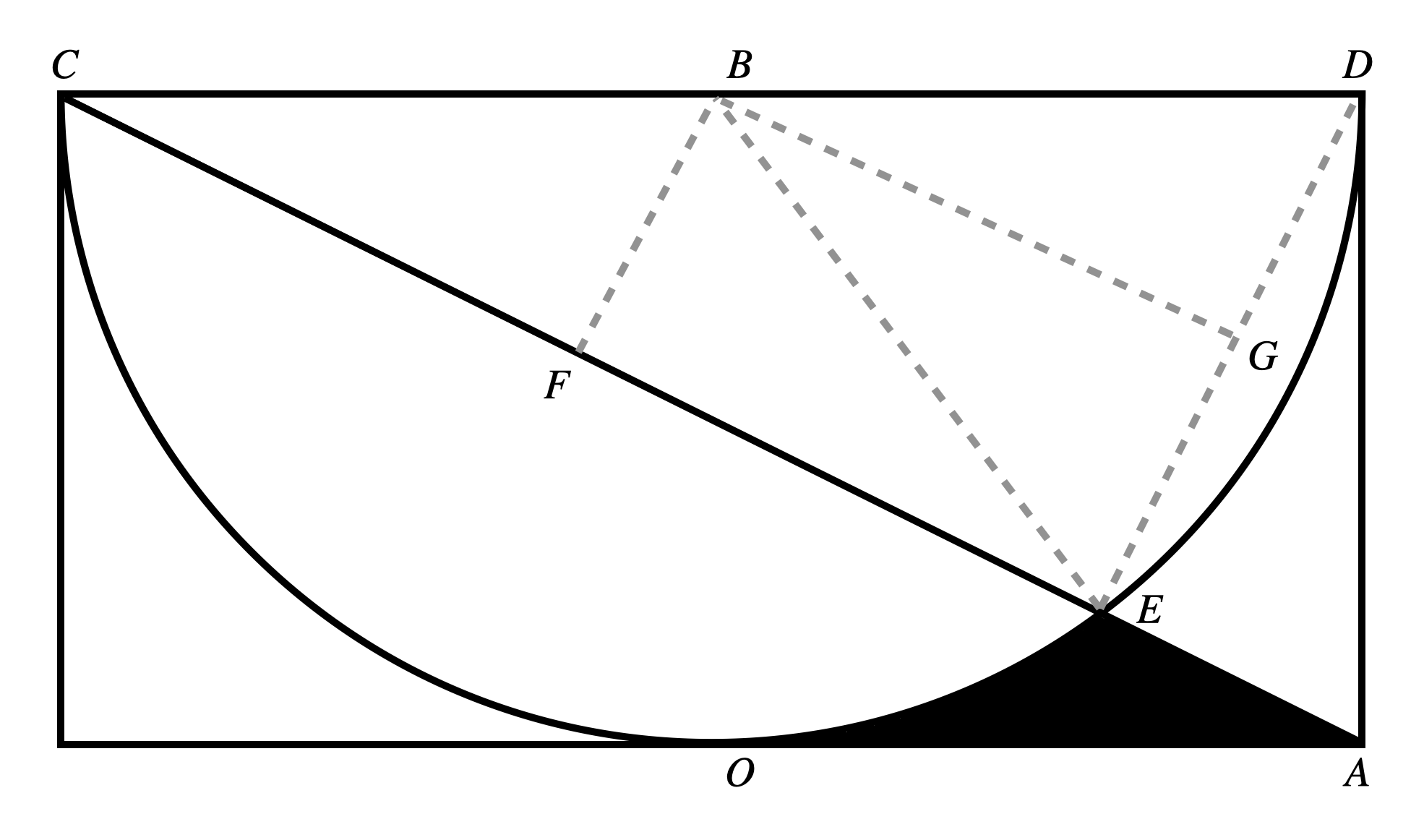$$\frac{32}{5}-4\pi+16 \arctan{\frac{1}{2}}$$

$$4\arctan{\frac{1}{2}}+2\arcsin{\frac{3}{5}}=\pi$$

## 总结与拓展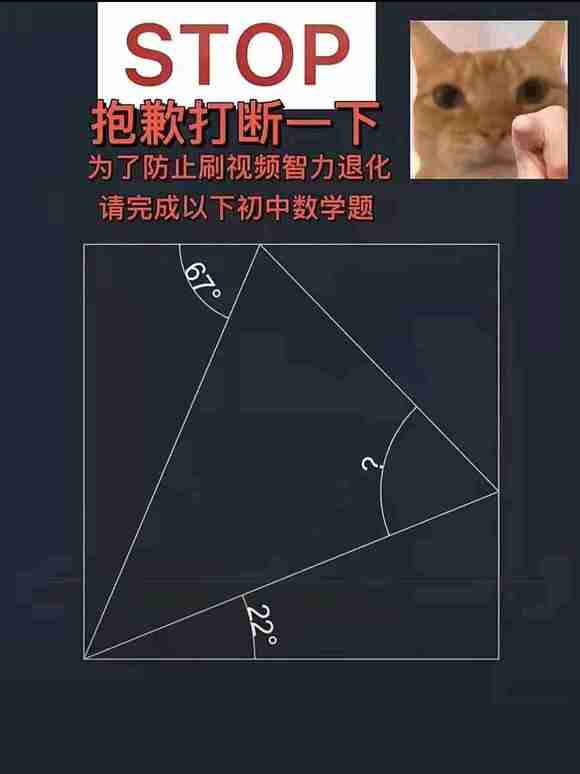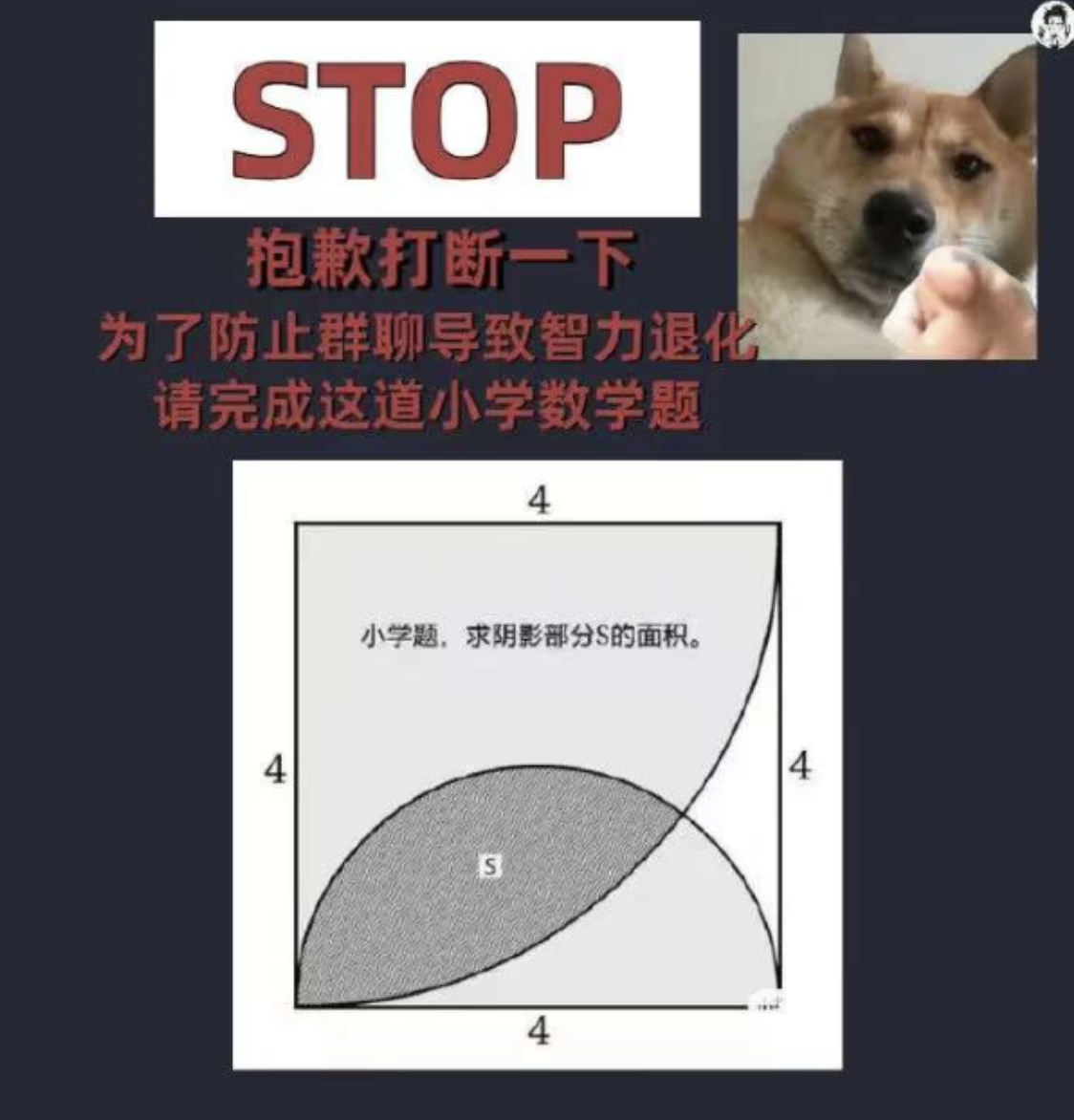转载规则

《这道小学六年级的数学题，恕我直言没几个人会做》 韦阳 采用 知识共享署名 4.0 国际许可协议 进行许可。
上一篇分析了B站所有的弹幕，原来大家都是这样的人

2021-12-18【白话模型量化系列二】量化感知训练

2021-12-17
目录